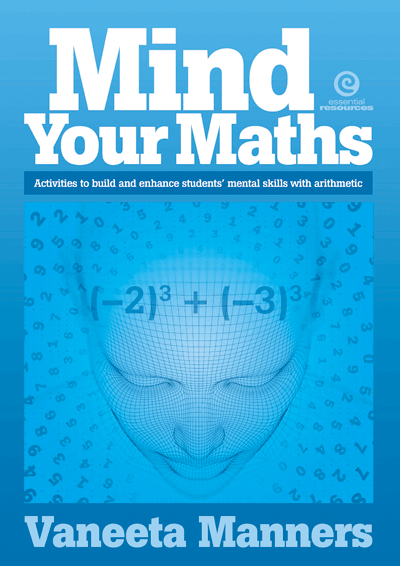-

## Activities to build and enhance students’ mental skills with arithmetic

ISBN: 9781776556137
Code: 51108While recognising that students need a calculator for certain topics such as trigonometry, the activities in this book deepen students’ insight into just how much arithmetic they can do successfully without one. They also set timeframes for completion so that students work on their speed as an essential component of effective mental arithmetic. Topics cover integers, fractions, decimals and percentages, emphasising the practice of operations such as powers of numbers and finding the square root and cube roots of fractions and decimals.

| Pages: 72 | Ages: 12-15

 or more each